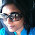Saturday, July 9, 2011

Cost to Fire Kiln

I ran a glaze firing and have calculated the costs on my new kiln. Always helpful to know what I am spending and where.

To calculate my costs....
My new Excel Select Fire 7 cubic. ft kiln is 11520 Watts or 11.52 kilowatts
Multiply kw x hrs fired. I also then apply a % based on that cone I fire to since I am not using the full power - about 50% for a bisque and 65% for glaze. This is an estimate- Multiply that x cost of kw (.21). Expensive electric on Long Island:(

Looks like this....

BISQUE
11.52 x .5 =5.76
5.76 x 7=40.32
40.32 x .21 = \$8.47

GLAZE
11520 /1000 = 11.52kw
11.52 x .65= 7.488
7.488 x 8.5 = 63.648
63.648 x .21 = \$13.37Brick Red and Black Rim

1 comment:

1.Hi,

So, if I understand it correctly, cost is \$8.47 to fire full load (7 cubic feet) for Bisque and \$13.37 for glaze.